# smoothdata2

Smooth noisy data in two dimensions

Since R2023b

## Syntax

``S = smoothdata2(A)``
``S = smoothdata2(A,method)``
``S = smoothdata2(A,method,window)``
``S = smoothdata2(___,nanflag)``
``S = smoothdata2(___,Name=Value)``
``[S,winsize] = smoothdata2(___)``

## Description

example

````S = smoothdata2(A)` smooths entries of `A` using a moving average. `smoothdata2` determines the moving window size from the entries in `A`.```

example

````S = smoothdata2(A,method)` smooths entries using the specified smoothing method. For example, `smoothdata2(A,"movmedian")` smooths by computing the median over each 2-D window of `A`.```

example

````S = smoothdata2(A,method,window)` specifies the size of the moving window. `window` specifies the number of preceding rows, succeeding rows, preceding columns, and succeeding columns to include in the window for the current element.```
````S = smoothdata2(___,nanflag)` specifies whether to omit or include `NaN` values in `A`. Use this option in addition to any of the input argument combinations in previous syntaxes. For example, `smoothdata2(A,"includenan")` includes all `NaN` values when smoothing. By default, `smoothdata2` ignores `NaN` values.```

example

````S = smoothdata2(___,Name=Value)` specifies additional parameters for smoothing using one or more name-value arguments. For example, `smoothdata2(A,SmoothingFactor=0.5)` adjusts the level of smoothing by specifying a factor that scales the window size that `smoothdata2` determines from the entries in A.```

example

````[S,winsize] = smoothdata2(___)` also returns the window description. If `window` is specified as an input, then the returned window description is the same as the specified window description.```

## Examples

collapse all

Create a matrix containing noise, and visualize the matrix along the `z`-dimension using a surface plot.

```A = peaks; rng(0,"twister") A = A + 0.5*randn(size(A)); surf(A)```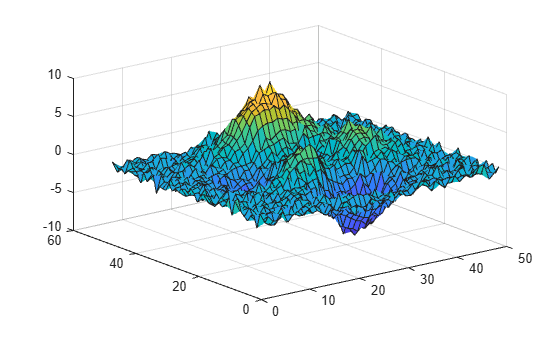Smooth the data using a moving average.

`B = smoothdata(A);`

Visualize the smoothed data.

`surf(B)`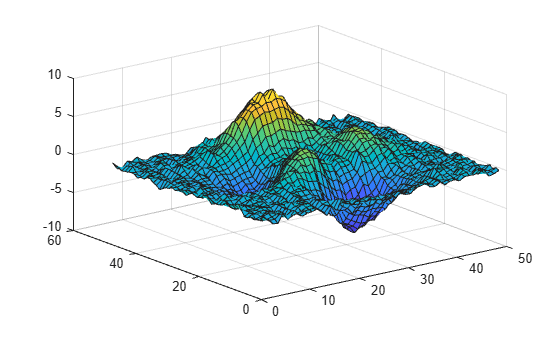In this example, explore and compare 2D smoothing methods. Each method has its own advantages and is suitable for different types of data and noise characteristics.

Create a matrix of data that is discretized and has sharp edges, and visualize the matrix using a surface plot.

```[bins,edges] = discretize(peaks,10); A = edges(bins); surf(A)```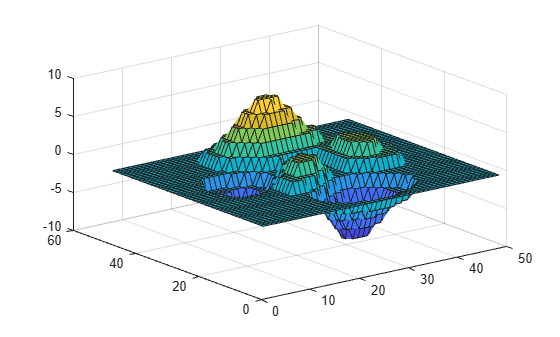Smooth the data using moving statistic smoothing methods.

```B = smoothdata2(A); B2 = smoothdata2(A,"movmedian");```

Compare the results of the smoothing methods by visualizing the smoothed data. The moving mean method is preferable to the moving median method for discretized 2D data with sharp edges. While the moving median method is robust against outliers, it may not adequately address the sharp edges in the data due to its focus on the middle value alone.

```tiledlayout(2,1) nexttile surf(B) title("Mean") nexttile surf(B2) title("Median")```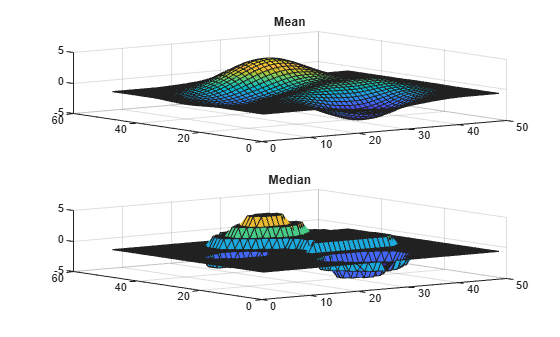Create a matrix with sharp variations occurring over an area smaller than the size of the smoothing window.

```x = 1:59; y = sin(x/5*pi); A2 = y + y'; figure surf(A2)```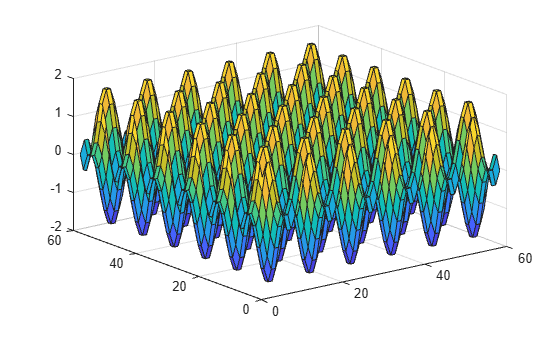Smooth the data usinga moving statistic smoothing method and a local regression smoothing method.

```B3 = smoothdata2(A2,"movmean",9); B4 = smoothdata2(A2,"gaussian",9);```

Compare the results of the smoothing methods by visualizing the smoothed data. The Gaussian smoothing method is better suited than the moving mean method for smoothing data with sharp variations due to its ability to preserve the sharp features while reducing noise. Unlike the moving mean method, which applies a simple average over the window, Gaussian smoothing uses a weighted average that assigns higher weights to nearby points.

```tiledlayout(2,1) nexttile surf(B3) title("Mean") nexttile surf(B4) title("Gaussian-Weighted Average")```Create a matrix containing noise, and visualize the matrix along the `z`-dimension using a heatmap chart.

```A = peaks(25); rng(0,"twister") A = A + 6*randn(size(A)); heatmap(A)```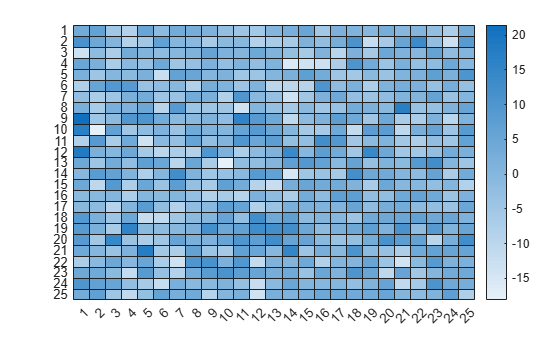Smooth the data by averaging over each 2-D window of `A`. Define the moving window as a 2-by-3 block of entries neighboring the current element.

`B = smoothdata2(A,"movmean",{2 3});`

Smooth the data over a larger moving window that is a 4-by-4 block of entries.

`B2 = smoothdata2(A,"movmean",4);`

Compare the results of the window sizes by visualizing the smoothed data.

```tiledlayout(2,1) nexttile heatmap(B,Title="2-by-3 Window") nexttile heatmap(B2,Title="4-by-4 Window")```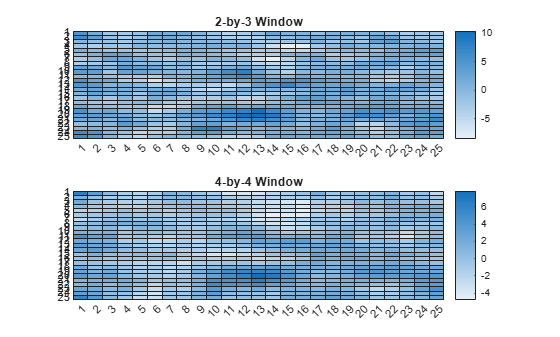Create a matrix containing noise, and visualize the matrix using a filled 2-D contour plot.

```A = peaks; rng(0,"twister") A = A + 4*randn(size(A)); contourf(A); colorbar```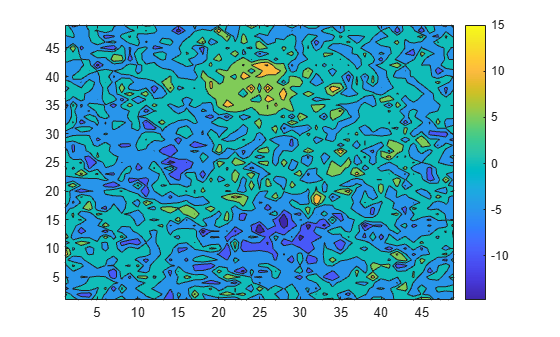Smooth the data by averaging over each 2-D window of `A`. `smoothdata2` determines the window size heuristically based on the input data and the default window size factor 0.25. Return the window size.

```[B,winsize] = smoothdata2(A); winsize```
```winsize=1×2 cell array {} {} ```

Smooth using a larger window size, resulting in more smoothing, by specifying a larger window size factor.

```[B2,winsize2] = smoothdata2(A,SmoothingFactor=0.5); winsize2```
```winsize2=1×2 cell array {} {} ```

Compare the results of the window size factors by visualizing the smoothed data.

```tiledlayout(2,1) nexttile contourf(B) title("Window Size Factor 0.25") colorbar nexttile contourf(B2) title("Window Size Factor 0.5") colorbar```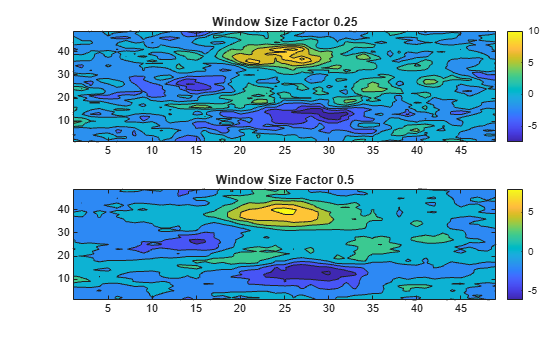Create a matrix of nonuniformly spaced data. Specify the sample points in vectors `xs` and `ys`.

```t = linspace(0,10,30); xs = cumsum(sin(pi/11*t)); ys = xs; A = sin(xs'/5)*cos(ys/5); rng(0,"twister") A = A+randn(size(A))*0.5;```

Visualize the matrix using a mesh surface plot.

```m = mesh(xs',ys,A,FaceAlpha=0.5); m.FaceColor = "flat"; view(0,90)```Smooth the data using linear regression over each 2-D window of `A`. Specify the window size, and specify the `x`-axis and `y`-axis locations of the data.

`B = smoothdata2(A,"loess",{[2 3],[4 3]},SamplePoints={xs,ys});`

Visualize the smoothed data.

```m2 = mesh(xs',ys,B,FaceAlpha=0.5); m2.FaceColor = "flat"; view(0,90)```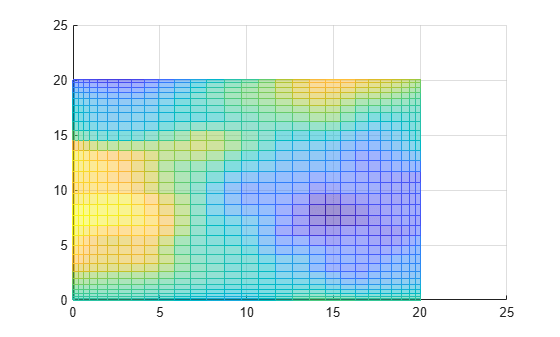## Input Arguments

collapse all

Input data, specified as a numeric array.

Complex Number Support: Yes

Smoothing method, specified as one of these values:

• `"movmean"` — Average over each 2-D window of `A`. This method is useful for reducing periodic trends in data.

• `"movmedian"` — Median over each 2-D window of `A`. This method is useful for reducing periodic trends in data when outliers are present.

• `"gaussian"` — Gaussian-weighted average over each 2-D window of `A`.

• `"lowess"` — Linear regression over each 2-D window of `A`. This method can be computationally expensive but results in fewer discontinuities.

• `"loess"` — Quadratic regression over each 2-D window of `A`. This method is slightly more computationally expensive than `"lowess"`.

• `"sgolay"` — Savitzky-Golay filter, which smooths according to a quadratic polynomial that is fitted over each 2-D window of `A`. This method can be more effective than other methods when the data varies rapidly.

Window size, specified as a positive integer or `duration` scalar, two-element cell array of positive integer or `duration` values, or two-element cell array of two-element vectors of nonnegative integer or `duration` values. `smoothdata2` defines the window relative to the sample points.

• When `window` is a positive integer scalar, then the window is a `window`-by-`window` block centered about the current element.

• If `window` is a two-element cell array of positive integers `{m n}`, then the window is an `m`-by-`n` block centered about the current element.

• If `window` is a two-element cell array of two-element vectors of nonnegative integers `{[bRow fRow] [bCol fCol]}`, then the window contains the row and column of the current element, the preceding `bRow` and succeeding `fRow` rows, and the preceding `bCol` and succeeding `fCol` columns.

When `A` is a timetable or `SamplePoints` contains `datetime` or `duration` values, `window` must be of type `duration`.

Example: `smoothdata2(A,"movmean",4)`

Example: `smoothdata2(A,"movmedian",{2 3})`

Example: `smoothdata2(A,"movmedian",{[0 2] [3 3]})`

Missing value condition, specified as one of these values:

• `"omitmissing"` or `"omitnan"` — Ignore `NaN` values in `A` when smoothing. If all elements in the window are `NaN`, then the corresponding elements in `S` are `NaN`. `"omitmissing"` and `"omitnan"` have the same behavior.

• `"includemissing"` or `"includenan"` — Include `NaN` values in `A` when smoothing. If any element in the window is `NaN`, then the corresponding elements in `S` are `NaN`. `"includemissing"` and `"includenan"` have the same behavior.

### Name-Value Arguments

Specify optional pairs of arguments as `Name1=Value1,...,NameN=ValueN`, where `Name` is the argument name and `Value` is the corresponding value. Name-value arguments must appear after other arguments, but the order of the pairs does not matter.

Example: `S = smoothdata2(A,SmoothingFactor=0.5)`

Sample points, specified as a two-element cell array of vectors of sample point values. The sample points in the first vector represent the data locations along the columns of `A`, and the sample points in the second vector represent the data locations along the rows of `A`. Both vectors must be sorted and must not contain duplicate elements. Sample points do not need to be uniformly spaced. If `A` is an `m`-by-`n` matrix, then the default value of `SamplePoints` is ```{1:n 1:m}```.

Moving windows are defined relative to the sample points. When the sample point vectors have data type `datetime` or `duration`, the moving window description must have type `duration`.

Window size factor, specified as a scalar ranging from 0 to 1. Generally, the value of `SmoothingFactor` adjusts the level of smoothing by scaling the heuristic window size. Values near 0 produce smaller moving window sizes, resulting in less smoothing. Values near 1 produce larger moving window sizes, resulting in more smoothing. In some cases, depending on the input data from which the heuristic window size is determined, the value of `SmoothingFactor` may not have a significant impact on the window size used by `smoothdata2`.

You cannot specify `SmoothingFactor` if you specify the `window` input argument.

Savitzky-Golay degree, specified as a nonnegative integer. You can only specify this name-value argument when `"sgolay"` is the specified smoothing method. The value of `Degree` corresponds to the degree of the polynomial in the Savitzky-Golay filter that fits the data within each window.

## Output Arguments

collapse all

Smoothed data, returned as a numeric array. `S` is the same size as `A`.

Window description, returned as a positive integer or `duration` scalar, a two-element cell array of positive integer or `duration` values, or a two-element cell array of two-element vectors of nonnegative integer or `duration` values.

If you specify `window` as an input argument, then `winsize` is the same as `window`. If you do not specify `window` as an input argument, then `winsize` is the scalar that `smoothdata2` determines heuristically based on the input data.

collapse all

### Moving Window Size

This table illustrates the window position along each dimension for the default uniformly spaced sample points vector ```[1 2 3 4 5 6 7]```.

Description

Window Size and Location

Sample Points in Window

Diagram

For a scalar window size, the leading edge of the window is included and the trailing edge of the window is excluded.

`window = 3`

Current sample point = 4

3, 4, 5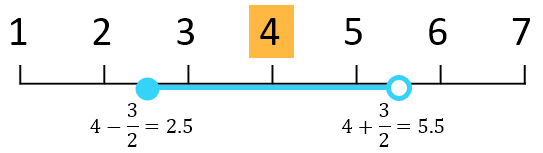`window = 4`

Current sample point = 4

2, 3, 4, 5For a vector window size, the leading edge and the trailing edge are included.

`window = [2 2]`

Current sample point = 4

2, 3, 4, 5, 6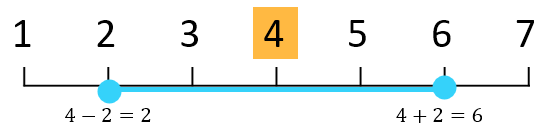For sample points near the endpoints of the input data, these moving statistic smoothing methods truncate the window so it begins at the first sample point or ends at the last sample point.

• `"movmean"`

• `"movmedian"`

• `"gaussian"`

`window = [2 2]`

Current sample point = 2

1, 2, 3, 4For sample points near the endpoints of the input data, these local regression smoothing methods shift the window to include the first or last sample point.

• `"lowess"`

• `"lowess"`

• `"sgolay"`

`window = [2 2]`

Current sample point = 2

1, 2, 3, 4, 5## Algorithms

When the window size for the smoothing method is not specified, `smoothdata2` computes a default window size based on a heuristic. For a smoothing factor τ, the heuristic estimates a moving average window size that attenuates approximately 100*τ percent of the energy of the input data.

## Version History

Introduced in R2023b